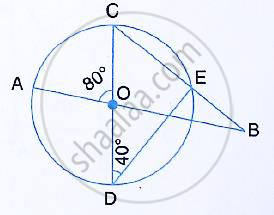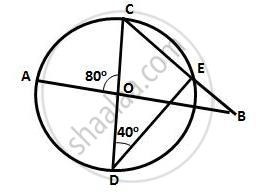Share

In the Figure Given Below, Ab and Cd Are Straight Lines Through the Centre O of a Circle. If ∠Aoc = 80° and ∠Cde = 40°, Find the Number of Degrees In: (I) ∠ Dce, (Ii) ∠Abc. - ICSE Class 10 - Mathematics

ConceptArc and Chord Properties - If Two Arcs Subtend Equal Angles at the Center, They Are Equal, and Its Converse

Question

In the figure given below, AB and CD are straight lines through the centre O of a circle. If ∠AOC
= 80° and ∠CDE = 40°, Find the number of degrees in:
(i) ∠ DCE, (ii) ∠ABC.Solution(i) Here, ∠CED =90°
(Angle in a semicircle is a right angle)
∴ ∠DCE = 90°  - ∠CDE = 90°  - 40°  = 50°
∴ ∠DCE = ∠OCB =50°
(ii) In ΔBOC,
∠AOC  = ∠OCB + ∠OBC
(Exterior angle of a Δ is equal to the sum of pair of interior opposite angles)
⇒ ∠OBC = 80°  - 50°  = 30°               [ ∠AOC = 80° ,given ]
Hence, ∠ABC = 30°

Is there an error in this question or solution?
Solution In the Figure Given Below, Ab and Cd Are Straight Lines Through the Centre O of a Circle. If ∠Aoc = 80° and ∠Cde = 40°, Find the Number of Degrees In: (I) ∠ Dce, (Ii) ∠Abc. Concept: Arc and Chord Properties - If Two Arcs Subtend Equal Angles at the Center, They Are Equal, and Its Converse.
S